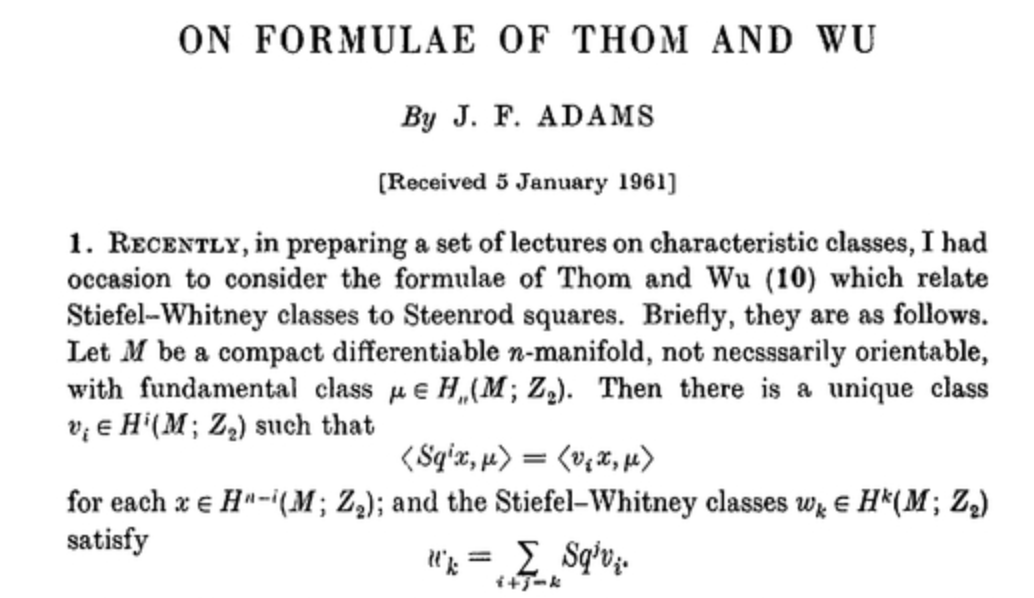# Who discovered this definition of Stiefel-Whitney classes?

I would define Stiefel-Whitney classes as the pullbacks of generators of $H^*(BO, \mathbb{Z}/2)$ under a classifying map, and I gather this is a pretty common definition.

However, the book "Characteristic classes" by Milnor-Stasheff contains a different definition, as eigenvalues'' for the Steenrod squares acting on the fundamental class of a manifold in its normal bundle. I want to attribute this construction to somebody for a paper, but I'm not sure what the correct attribution is. Who is the discoverer of this definition?

• That is not a definition at all, as the generators" does not define elements of a ring. Dec 19, 2016 at 21:52
• Sorry, I purposefully did not say "the generators" but maybe should have said "certain generators", which I realize is not actually a definition either, but is probably enough indication of what I'm referring to. Dec 19, 2016 at 22:13
• @OscarRandal-Williams I think he meant the homogeneous polynomial generators, which I believe in this case is in fact a characterization of the elements of $H^*(BO;\mathbb{Z}/2)$ we are talking about Dec 19, 2016 at 22:32
• Well, you give me too much credit. What I actually have in my head is a specific choice of generator labelled $w_n$, corresponding to a specific symmetric polynomial under the embedding $H^*(BO(n)) \hookrightarrow H^*(BO(1)^n)$. I lazily decided not to be more precise, thinking that nobody would care, but that seems to have been a wrong presumption. Dec 19, 2016 at 22:47
• @user84144: Is this really a definition? The only way I can think of showing that map i) is injective, and ii) hits the symmetric polynomials, needs me to already have a definition of Stiefel-Whitney classes and have proved the sum formula for them. Dec 19, 2016 at 23:42

For the relation $Sq(U) = \Phi(w)$, where $Sq$ is the total Steenrod squaring operation, $U$ is the Thom class, $\Phi$ is the Thom isomorphism and $w$ is the total Stiefel-Whitney class, I would cite Rene Thom's 1951 thesis, published as "Espaces fibres en spheres et carres de Steenrod" in Ann. Sci. Ecole Norm. Sup. (3) 69 (1952). In the introduction he explains that he found the results for manifolds embedded in Euclidean space, and that Henri Cartan then showed him how to obtain the general case. These results were first announced by Thom in two Comptes Rendus Acad. Sc. notes (t. 230, 1950, p. 427 and p. 507). Wen-Tsun Wu's formula $Sq(v)=w$ in the case of the tangent bundle of a closed manifold is not the same result. It was announced in the same volume of Comptes Rendus (p. 508 and p. 918). Its proof depends on the results of Thom.

The earliest reference for the connection between Stiefel–Whitney classes and Steenrod squares seems to be Wen-tsün Wu, Classes caractéristiques et i-carrés d'une variété, C.R. Acad. Sci. Paris 230, 508–511 (1950), followed a few years later by René Thom, Quelques propriétés globales des variétés différentiables, Comment. Math. Helv. 28, 17–86 (1954).